## Analysing Economic News Project report

Note: The edits to the page are being made in the reverse chronological manner.The project has, apparently, come to a formal end with the final BTP presentation and reports made and submitted. They can be found here:

The system is now online with the following GUI: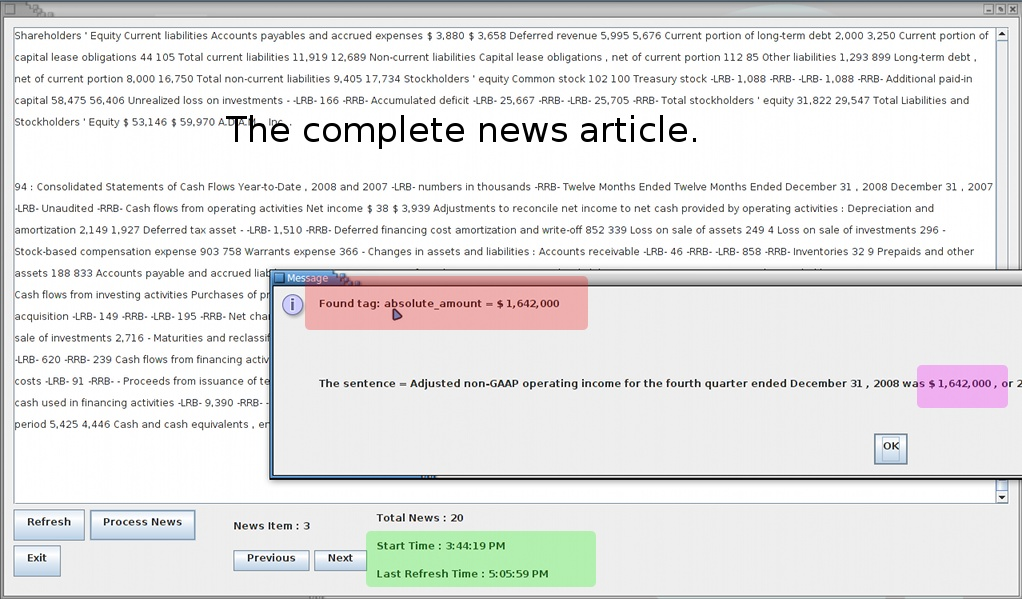The buttons are explained below:

• Process News : Scans the news for tags. The pop-up box cycles through all the tags found in the news articles sentence by sentence.
• Refresh : Fetches any new news articles using the Yahoo RSS feed.
• Next / Prev : Cycles through the news articles that have been fetched.

However, even while using the PCFG parser, the performance of the system on my machine (1.2 GHz, 2 Gb RAM) raises a few doubts (the green section reports the time it took to parse 20 news articles.) The Factored Parser merrily takes hours to process the same 20 articles. However, the figures depend heavily on the nature of the news articles too. For example, the news articles visible on the screen-shot had 94 lines that needed to be parsed.

The PathFollower, which searches for tree structures is complete, with the following example output:

```In File : 2 for predicate : be and path/tag: VBD(up)VP(down)NP(down)NP/absolute_amount there are 3 possibilities.
\$ 1,783,000

an increase of \$ 303,000

20 % from net income of \$ 1,480,000
```

The file in which this search was made was 2 , the predicate was be and the element being searched for was absolute_amount . The path predicted for it was: VBD(up)VP(down)NP(down)NP and in the statement, three possible fits to this tree path were found, which are listed.

The original sentence was:

```(ROOT
(S
(S
(NP (NNS PALOS))
(VP (VBZ VERDES)
(NP
(NP
(NP (NNS ESTATES))
(, ,)
(NP (NNP Calif.))
(: --))
(PRN (-LRB- -LRB-)
(-RRB- -RRB-)))))
(: --)
(S
(NP
(NP (NNP Malaga) (NNP Financial) (NNP Corporation))
(PRN (-LRB- -LRB-)
(NP (NN OTCBB) (: :) (NN MLGF))
(, -)
(NP (NNP News))
(-RRB- -RRB-))
(, ,)
(NP
(NP (DT the) (NN parent) (NN company))
(PP (IN of)
(NP (NNP Malaga) (NNP Bank) (NNP FSB))))
(, ,))
(NP-TMP (NN today))
(VP (VBD reported)
(SBAR (IN that)
(S
(NP
(NP (JJ net) (NN income))
(PP (IN for)
(NP
(NP (DT the) (NN quarter))
(VP (VBN ended)
(NP-TMP (NNP September) (CD 30) (, ,) (CD 2008))))))
(VP (VBD was)
(NP
(NP (\$ \$) (CD 1,783,000))
(, ,)
(NP
(NP (DT an) (NN increase))
(PP (IN of)
(NP (\$ \$) (CD 303,000))))
(CC or)
(NP
(NP (CD 20) (NN %))
(PP (IN from)
(NP
(NP (JJ net) (NN income))
(PP (IN of)
(NP (\$ \$) (CD 1,480,000)))))))
(PP (IN for)
(NP
(NP (DT the) (NN quarter))
(VP (VBN ended)
(NP-TMP (NNP September) (CD 30) (, ,) (CD 2007))))))))))
(. .)))
```

or in words:

PALOS VERDES ESTATES , Calif. - -LRB- BUSINESS WIRE -RRB- - Malaga Financial Corporation -LRB- OTCBB : MLGF - News -RRB- , the parent company of Malaga Bank FSB , today reported that net income for the quarter ended September 30 , 2008 was \$ 1,783,000 , an increase of \$ 303,000 or 20 % from net income of \$ 1,480,000 for the quarter ended September 30 , 2007 .

The information thus extracted is not perfect, but beyond this, it should be relatively easy to fill out frames using the standard syntax rules.

After annotations had been dealt with, figuring out paths from elements to other elements was comparatively easy. Though the Parser API is a little finicky here. It only gives me paths of 'objects' which are subtrees of the original tree, not the 'subtrees' by content. A typical issue of using == equivalence instead of .equals() . It understandably saves some computation on regular searches. However, owing to it, my code has some overhead.

• The complete sentence: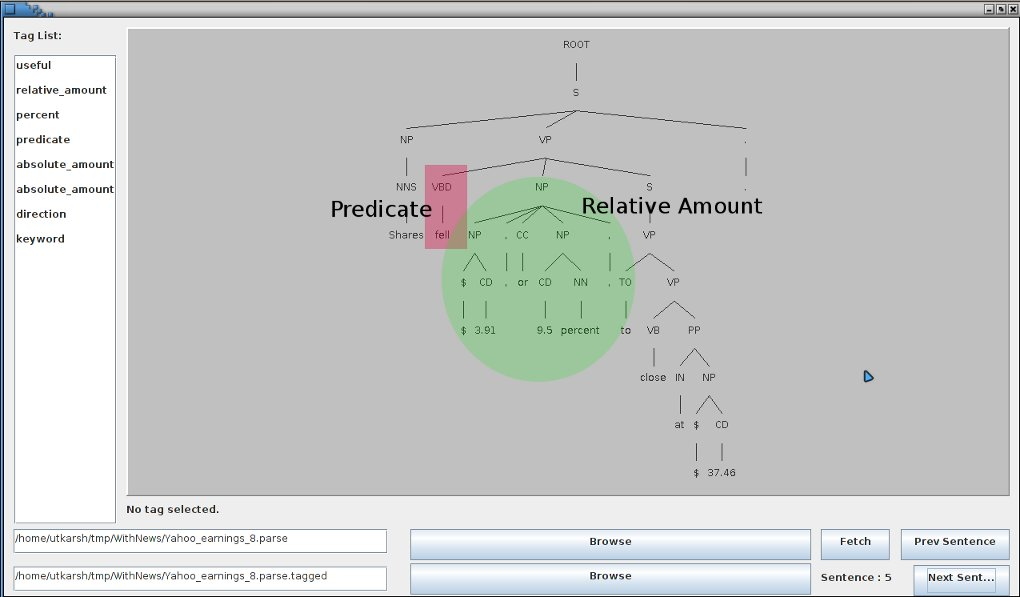• The path from the predicate to the rel amt :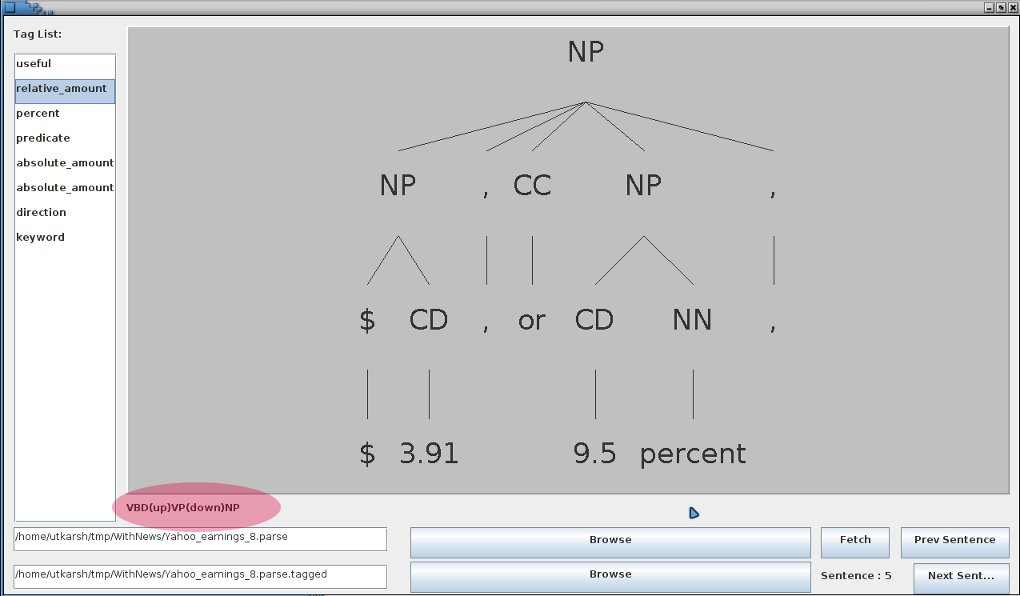It can be seen that in the actual tree, we need to start from the VBD node, then ascend to VP and then descend to NP which is the relative amount.

Finally, the annotating task has come to a close, and it required some tricks I had not thought of before. Anyways, all is well that ends well.

• Actual sentence: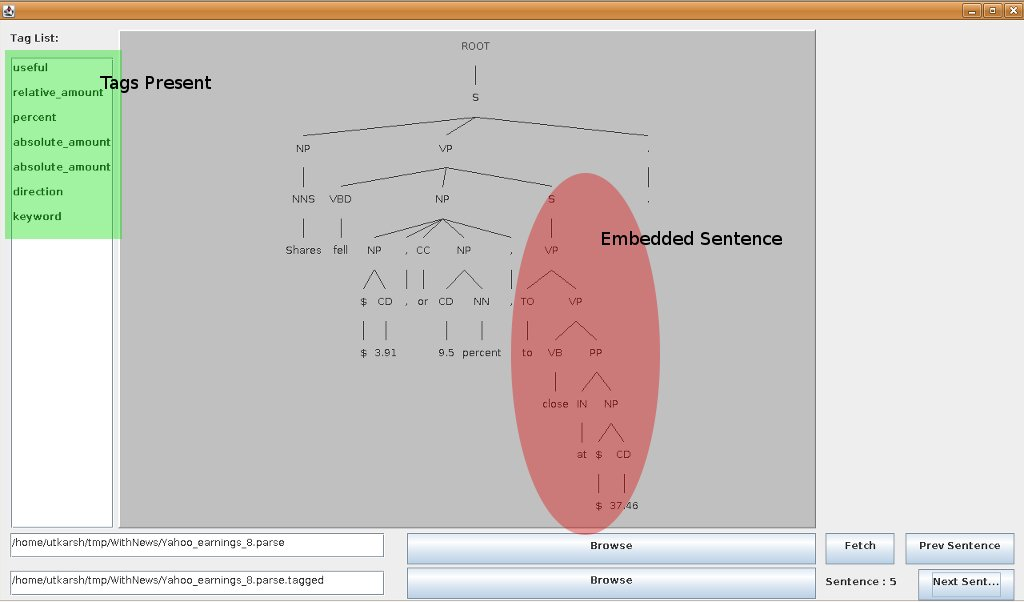• The useful part: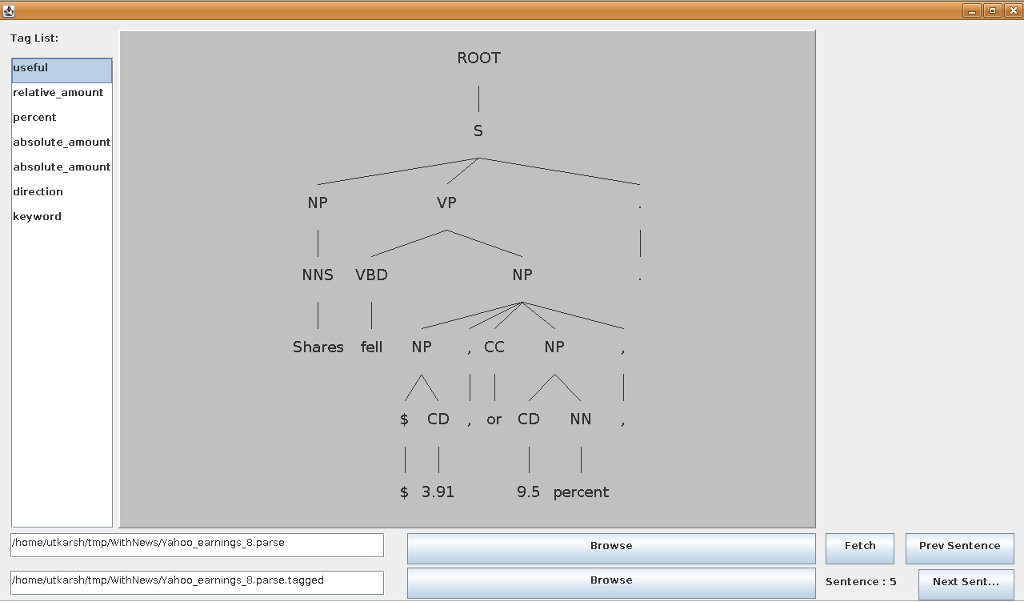• The Relative Amount part: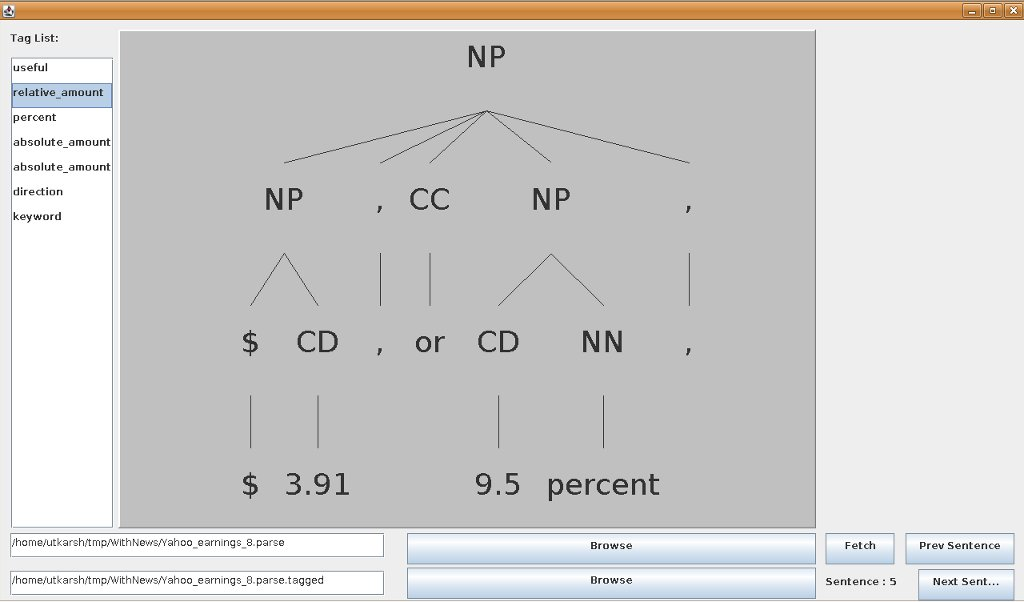• The Time part (from a difference sentence):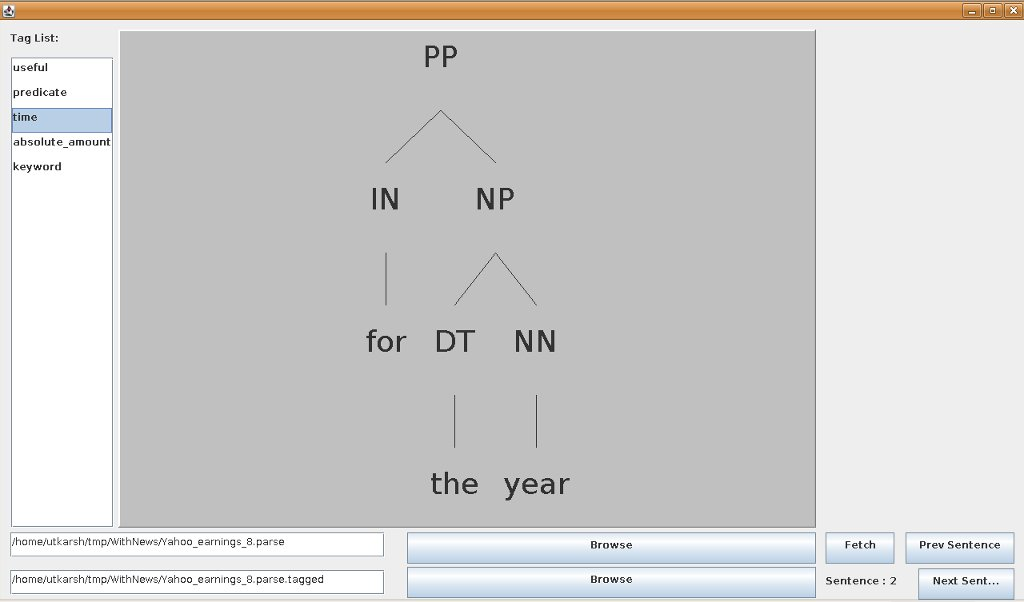Note that the sentence chosen in this case has a S embedded in it. And hence, the expression that I had initially thought of:

```{((( __ << ( word1 .. word2 .. word3 .. word4 )) ,, preceding_word) .. succeeding_word)}
```

would not work in this case because the preceding word and/or the succeeding word may fall in separate segments. Also, there were some sentences which do not start with a S immediately under the ROOT node. For those, manual renaming of the node had to be done as a work around.

Edit:

However, it seems that even the new Tregex fails under some (actually very obvious) cases. Hence, the search has been made a little stricter by using the following Tregex in that order:

```( __ << ( word1 . word2 . word3 . word4 ))
(( __ << ( word1 . word2 . word3 . word4 )) , preceding_word)
(( __ << ( word1 . word2 . word3 . word4 )) . succeeding_word)
((( __ << ( word1 . word2 . word3 . word4 )) , preceding_word) . succeeding_word)
```

...and I had thought that making the regular expression to extract sequence of words from xml tagged sentences would be easy. I don't think I was ever that wrong.

(Maybe leaving that sweets box in the sun to get rid of the ants, thereby causing it to be eaten by the crows would count, but that is not the point.)

Anyways, this is what my code looks like now:

``` 	// Pattern pat = Pattern.compile("\\b(.*?)\\b<" + tag + ">" + "(.*?)" + "</" + tag + ">[\\s]*(.*?)\\b");
// Pattern pat = Pattern.compile("(\\b.*?\\b)?[\\s]*<" + tag + ">[\\s]*(.*?)[\\s]*</" + tag + ">[\\s]*(\\b.+?\\b)?");
// Pattern pat = Pattern.compile("(\\b[^\\s>]+?\\b)?[\\s]*(<[^<]*?>)*[\\s]*<" + tag + ">[\\s]*(.*?)[\\s]*</" + tag + ">[\\s]*(<[^>]*?>)*(\\b.+?\\b)?");
// Pattern pat = Pattern.compile("(\\b?[^\\s>]+?)?[\\s]*(<[^<]*?>)*[\\s]*<" + tag + ">[\\s]*(.*?)[\\s]*</" + tag + ">[\\s]*(<[^>]*?>)*([^\\s<]+?\\b?)?");
// This regular expression is the hard work of hours and is still not perfect.
// May lord bless the undead and those who promote Unit testing.
Pattern pat = Pattern.compile("(\\b?[^\\s>]+)?[\\s]*(<[^<]*?>)*[\\s]*<" + tag + ">[\\s]*(.*?)[\\s]*</" + tag + ">[\\s]*(<[^>]*?>)*[\\s]*([^\\s<]+\\b?)?");
```

As of now, they can read the following sentences (at least):

```  "<name>BBC</name> <predicate>is</predicate>.",
"BBC's shares <predicate><direction>fell</direction></predicate>.",
"The drop took place in <time>the third quarter</time>.",
"<useful>Analysts polled by Thomson Reuters <predicate>expect</predicate> , on average ,
<keyword>revenue</keyword> of <absolute_amount>\$ 11.65 billion</absolute_amount><time> for the year</time> .</useful>"
```

It will not be long before I will be able to analyze sentences' structures annotated with their phrasal structures.

As of now, Ramnik and I are working on the Interactive Broker's trading Olympiad and trying to make strategies made using Ptolemy work with the IB Java API.

Edit:

It grew worse:

```   // Pattern pat = Pattern.compile("(\\b?[^\\s>]+)?[\\s]*(<[^<]*?>)*[\\s]*<" + tag + ">[\\s]*(.*?)[\\s]*</" + tag + ">[\\s]*(<[^>]*?>)*[\\s]*([^\\s<]+\\b?)?");
Pattern pat = Pattern.compile("(\\b?[^\\s>]+)?[\\s]*([\\s]*<[^<]*?>)*[\\s]*<" + tag + ">[\\s]*(.*?)[\\s]*</" + tag + ">[\\s]*(<[^>]*?>[\\s]*)*[\\s]*([^\\s<]+\\b?)?");
```

After analysis of various news articles, there are some templates which are extremely rare, e.g. the ``reference'' in the relative amount was filled only in 1 sentence out of about 30 useful and deceptive sentences skimmed out of 20 news articles. Hence, the tagging guidelines will need a little modification.

The predicate list (unstemmed) was:

```	rose
fell
was
tumbled
saw
dropped
reported
expect
posted
cut
expect
closed
```

which goes to show in its own way what the state the economy is in. It also presents an unforeseen problem; that of unrecognized predicates.

The analysis of the tagged sentences' parse-tree structures, which promises some insights, will ensue. To extract the node governing the tagged group of words in a parse tree, I will be utilizing Tsurgeon and the following Tregex pattern quite liberally:

```{((( __ << ( word1 .. word2 .. word3 .. word4 )) ,, preceding_word) .. succeeding_word)}
```

As an afterthought, if it was not for TregexPatterns, I would be using Perl instead of rather cumbersome Java for the task.

The strategy for summarization of news has also changed. It has come to our notice that the Lexical parser at times is capable of performing much better parses than the PCFG parser on the sentences which are useful. However, as parsing is the time consuming process, the summarization cannot be done after parsing. Hence, keyword based summarization will be done and then Lexical parsing of select sentences will follow.

The following images show a sample difference between the PCFG and the Factored parses:

Both trees: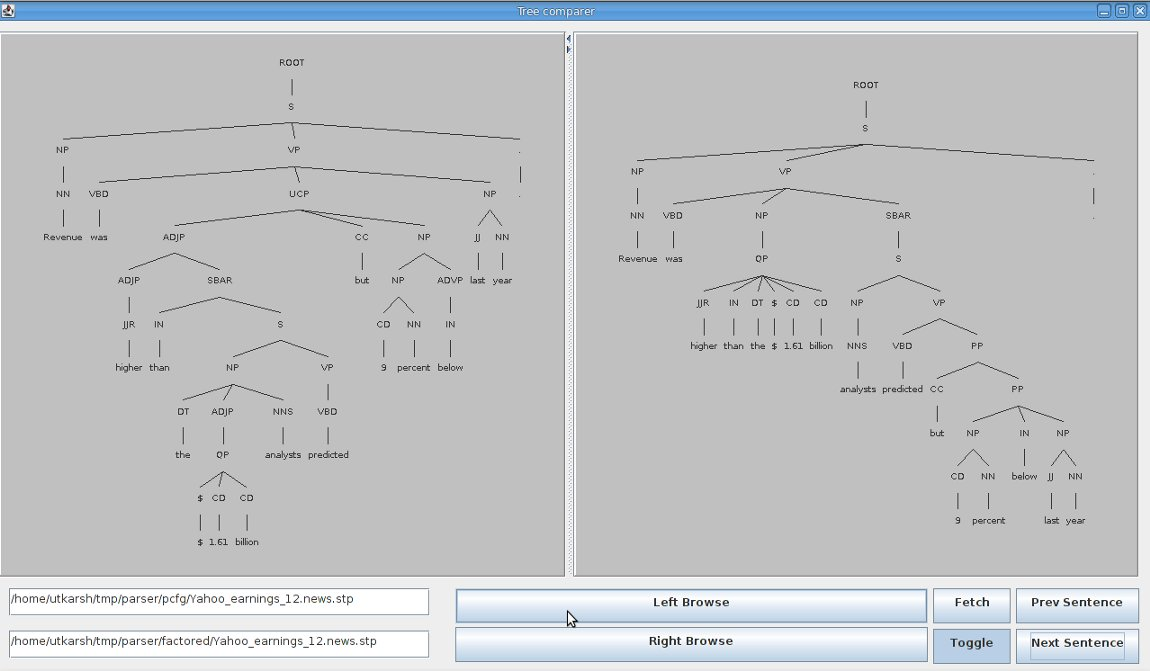The work has progressed further that what has been explained in the report , but has been put on a temporary hold as my project partner, Ramnik Arora is on a short vacation and I am putting in my application to various universities. When last heard of, the following GUI was being used for manual tagging of sentences to act as a sample learning set. (09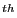December, '08), and the strategy for simplistic tagging was this.

Also, I am not using the Stanford annotator because it does not ensure that the tagging done will have proper syntactic structure, i.e., the tags may not contain exactly a subtree of the parse tree.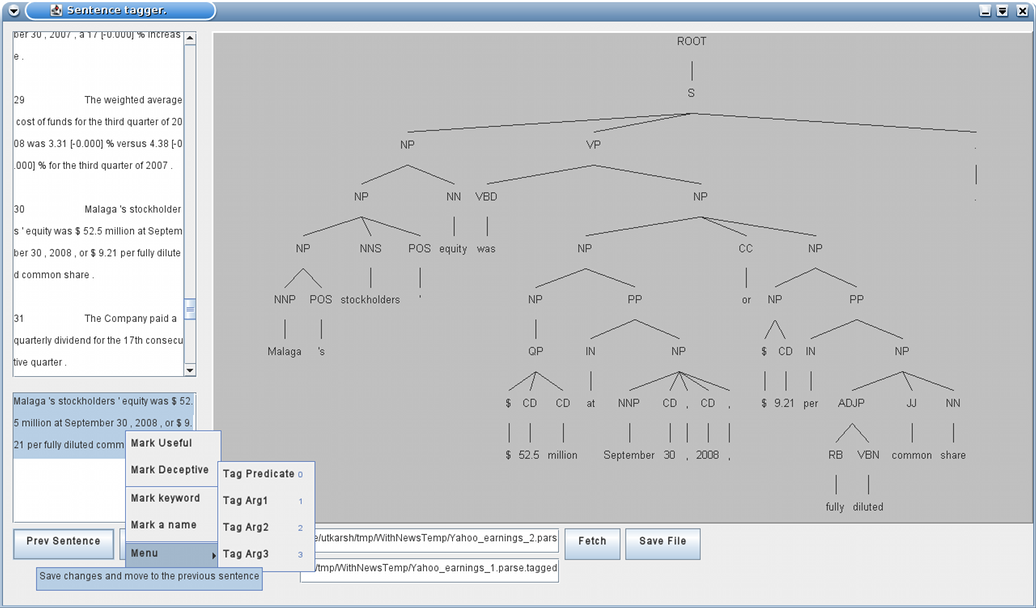musically_ut 2014-04-03